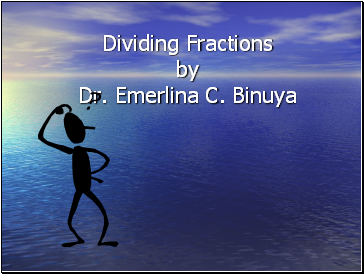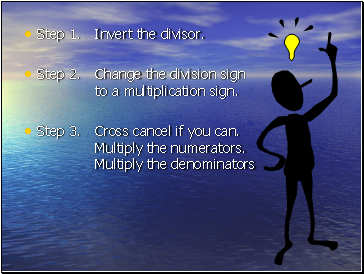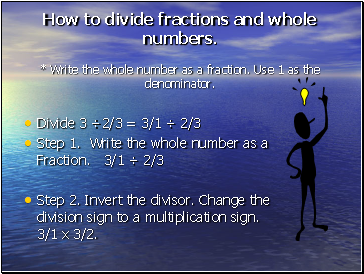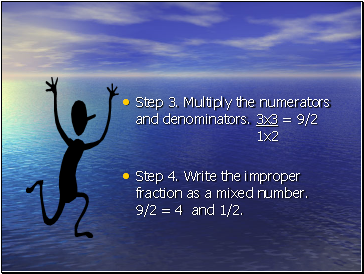# Dividing FractionsPage 1

#### WATCH ALL SLIDES

Slide 1## Dividing Fractions

by Dr. Emerlina C. Binuya

Slide 2Step 1. Invert the divisor.

Step 2. Change the division sign to a multiplication sign.

Step 3. Cross cancel if you can. Multiply the numerators. Multiply the denominators

Slide 3## How to divide fractions and whole numbers.

* Write the whole number as a fraction. Use 1 as the denominator.

Divide 3 ÷2/3 = 3/1 ÷ 2/3

Step 1. Write the whole number as a Fraction. 3/1 ÷ 2/3

Step 2. Invert the divisor. Change the division sign to a multiplication sign. 3/1 x 3/2.

Slide 4Step 3. Multiply the numerators and denominators. 3x3 = 9/2 1x2

Step 4. Write the improper fraction as a mixed number. 9/2 = 4 and 1/2.

Slide 5Reminder: To change an improper fraction to a mixed number, divide the numerator by the denominator. Put the remainder over the denominator.